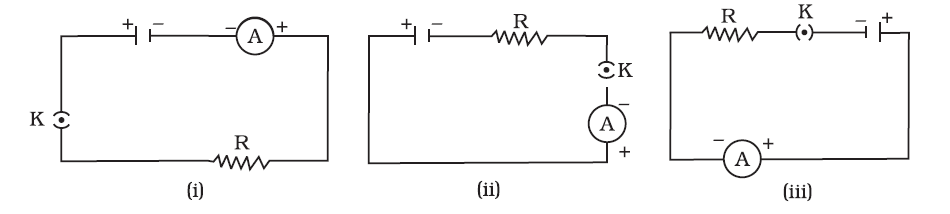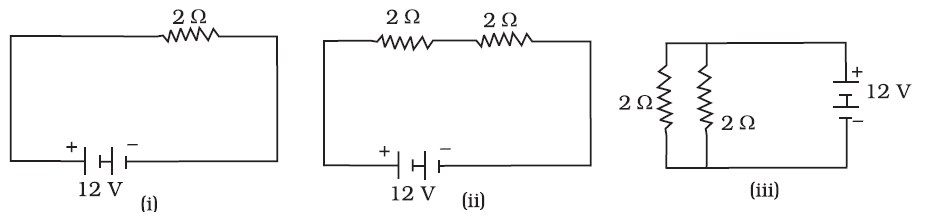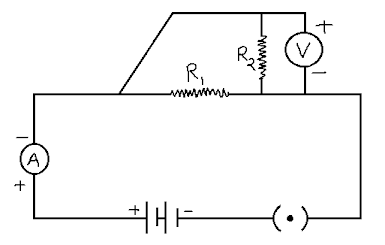Electricity test - quiz
Online test on Electric Circuits - 10 Questions
class *
Study the circuit and answer Q11. A cell, a resistor, a key and ammeter are arranged as shown in the circuit diagrams. The current recorded in the ammeter will be *
1 point
Study the following circuit and answer Q22. In the above circuits, heat produced in the resistor or combination of resistors connected to a 12 V battery will be *
1 point
Study the circuit given below and answer Q33. Which of the circuit components in the above circuit diagram are connected in parallel? *
1 point